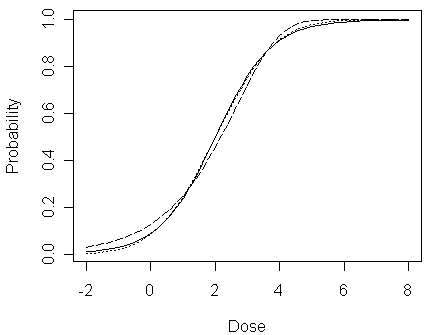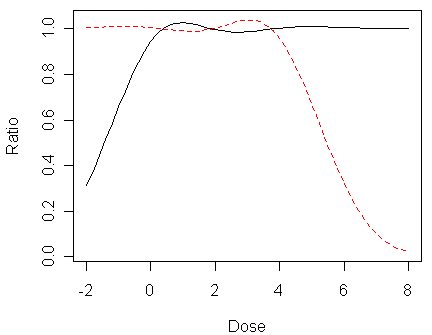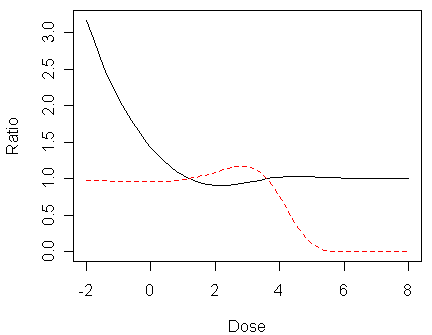Bliss (1935) analyzed some data on the number of insects dying at different levels of insecticide concentration. Let us first read into R the data and take a look of it:

> bliss

1    2    28    0

2    8    22    1

3   15    15    2

4   23     7    3

5   27     3    4

ˇ@

We now fit the binomial GLM to the data under the three link functions --- logit, probit, and complementary log-log:

> modl <- glm(cbind(dead,alive) ~ conc, family=binomial, data=bliss)
ˇ@

We start by considering the fitted values of probability px:

> fitted(modl) # or use the command "predict(modl,type="response")"

1          2          3          4          5

0.08917177 0.23832314 0.50000000 0.76167686 0.91082823

An alternative way to obtain these values is to use linear predictor, hx:

> coef(modl)+coef(modl)*bliss\$conc

 -2.323790e+00 -1.161895e+00  4.440892e-16  1.161895e+00  2.323790e+00

The values of linear predictor can also be obtained from:

> modl\$linear.predictors # or use the command "predict(modl)"

1             2             3             4             5

-2.323790e+00 -1.161895e+00  4.440892e-16  1.161895e+00  2.323790e+00

The fitted values of probability are then:

> ilogit(modl\$linear.predictors)

1          2          3          4          5

0.08917177 0.23832314 0.50000000 0.76167686 0.91082823

Notice the need to distinguish between predictions in the scale of the response (i.e., px) and the link (i.e., hx).

ˇ@

Now, let us compare the logit, probit, and complementary log-log fits:

> cbind(fitted(modl),fitted(modp),fitted(modc))

[,1]       [,2]      [,3]

1 0.08917177 0.08424186 0.1272700

2 0.23832314 0.24487335 0.2496909

3 0.50000000 0.49827210 0.4545910

4 0.76167686 0.75239612 0.7217655

5 0.91082823 0.91441122 0.9327715

These are not very different, but now look at a wider range -2<concentration<8:

> x <- seq(-2,8,0.2)
> pl <- ilogit(modl\$coef+modl\$coef*x)
> pp <- pnorm(modp\$coef+modp\$coef*x)
> pc <- 1-exp(-exp((modc\$coef+modc\$coef*x)))
> plot(x,pl,type="l",ylab="Probability",xlab="Dose")
> lines(x,pp,lty=3)
> lines(x,pc,lty=5)In the figure, logit fit is shown by a solid line, probit fit a dotted line, complementary log-log a dashed line. We can see that:

• when 0.2 <  px <0.8, the three lines do not seem very different (both on their differences and on their ratios)

• however, when px is close to 0 or 1, although their differences are still small, their ratios differ substantially as shown in the following two figures

• This is problematic since the concentration in the dataset falls in the range: [0, 4], which indicates it would be difficult to distinguish between these link functions using the data

> matplot(x,cbind(pp/pl,(1-pp)/(1-pl)),type="l",xlab="Dose",ylab="Ratio")In the figure, the lower tail ratio of probit to logit probabilities (i.e., px,probit/px,logit) is given by the solid line, the upper tail ratio (i.e., (1-px,probit)/(1-px,logit)) is given by the dashed line.

> matplot(x,cbind(pc/pl,(1-pc)/(1-pl)),type="l",xlab="Dose",ylab="Ratio")In the figure, the lower tail ratio of complementary log-log to logit probabilities is given by the solid line, the upper tail ratio is given by the dashed line.